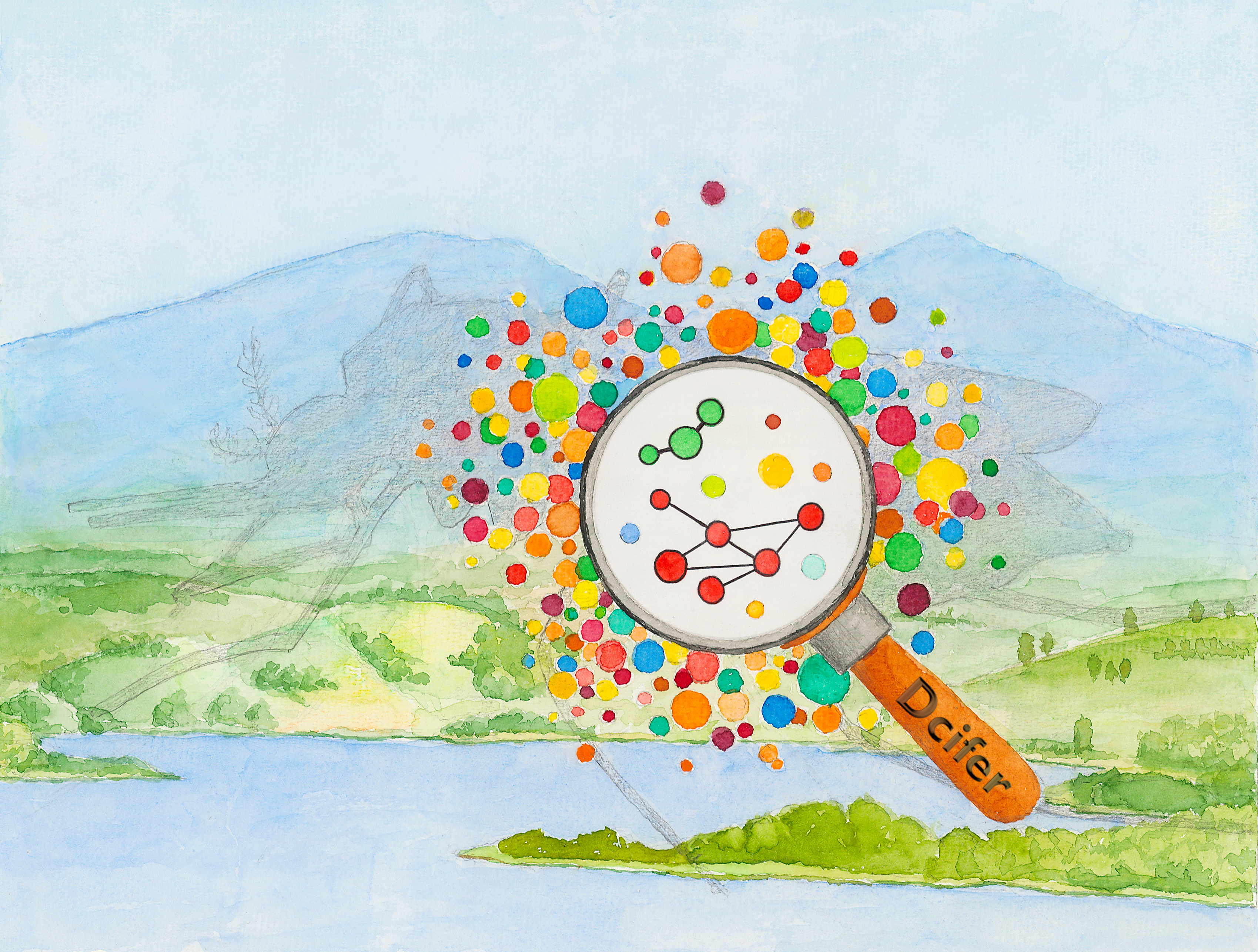Package dcifer (Distance for Complex Infections: Fast Estimation of Relatedness) calculates genetic distance between polyclonal infections by estimating relatedness from biallelic and multiallelic data . In addition to estimates, the package provides a likelihood function and statistical inference. Functions for reading and reformatting data, performing preparatory steps, and visualizing the results are also included. We will illustrate the analysis process using microhaplotype data from two health facilities in Maputo and Inhambane provinces of Mozambique .

You can install the released version of dcifer from CRAN with:

install.packages("dcifer")

and the development version from GitHub with:

# install.packages("remotes")
remotes::install_github("EPPIcenter/dcifer")
# or, to include a vignette:
remotes::install_github("EPPIcenter/dcifer", build_vignettes = TRUE)
library(dcifer)
help(package = "dcifer")
vignette(package = "dcifer", "vignetteDcifer")
pardef <- par(no.readonly = TRUE)

## Read and reformat data, estimate COI and population allele frequencies

Dcifer relatedness estimation requires sample data in a specific format as well as estimates of complexity of infection (COI) and population allele frequencies. Here is an example of some preparation steps using Mozambique dataset. First, read in original data stored in a .csv file in a long format.

sampleID locus allele clinic province
8025874217 t1 HB3.0 Inhassoro Inhambane
8025874217 t1 D10–D6–FCR3–V1-S.0 Inhassoro Inhambane
8025874217 t20 U659.0 Inhassoro Inhambane

The name of the file is the first argument to the function that reads and reformats the data:

sfile <- system.file("extdata", "MozParagon.csv", package = "dcifer")
dsmp <- readDat(sfile, svar = "sampleID", lvar = "locus", avar = "allele")
str(dsmp, list.len = 2)
#> List of 52
#>  $8025874217:List of 87 #> ..$ t1  : Named num [1:5] 1 1 0 0 0
#>   .. ..- attr(*, "names")= chr [1:5] "D10--D6--FCR3--V1-S.0" "HB3.0" "t1.0" "t1.1" ...
#>   ..$t10 : Named num [1:4] 1 0 0 0 #> .. ..- attr(*, "names")= chr [1:4] "D10--D6--HB3.0" "t10.0" "t10.2" "U659.0" #> .. [list output truncated] #>$ 8025874237:List of 87
#>   ..$t1 : Named num [1:5] 0 0 0 0 1 #> .. ..- attr(*, "names")= chr [1:5] "D10--D6--FCR3--V1-S.0" "HB3.0" "t1.0" "t1.1" ... #> ..$ t10 : Named num [1:4] 1 0 0 0
#>   .. ..- attr(*, "names")= chr [1:4] "D10--D6--HB3.0" "t10.0" "t10.2" "U659.0"
#>   .. [list output truncated]
#>   [list output truncated]

Or, to reformat an R data frame (dlong) containing the same dataset:

dsmp <- formatDat(dlong, svar = "sampleID", lvar = "locus", avar = "allele")
# optionally, extract location information
meta <- meta[match(names(dsmp), meta$sampleID), ] # order samples as in dsmp Next, estimate COI for all the samples - here we use naive estimation, first ranking loci of a sample by the number of detected alleles, and then using a locus with a prescribed rank (lrank) to determine COI: lrank <- 2 coi <- getCOI(dsmp, lrank = lrank) Finally, estimate population allele frequencies, adjusting for COI: afreq <- calcAfreq(dsmp, coi, tol = 1e-5) str(afreq, list.len = 2) #> List of 87 #>$ t1  : Named num [1:5] 0.4239 0.2808 0.1116 0.0422 0.1415
#>   ..- attr(*, "names")= chr [1:5] "D10--D6--FCR3--V1-S.0" "HB3.0" "t1.0" "t1.1" ...
ord       <- order(factor(meta$province, levels = provinces)) dsmp <- dsmp[ord] coi <- coi[ ord] dres <- ibdDat(dsmp, coi, afreq, nr = 1e3)  ## Visualize the results When pairwise relatedness is calculated within a single dataset, ibdDat returns triangular matrices. For plotting, we can make them symmetric. Then significantly related pairs can be outlined in either or both triangles. Display the results for unsorted samples, with sample ID’s written on the margins. Label colors correspond to locations (health facilities): par(mar = c(3, 3, 1, 1)) alpha <- 0.05 # significance level dmat <- dres0[, , "estimate"] # create symmetric matrix dmat[upper.tri(dmat)] <- t(dmat)[upper.tri(t(dmat))] # determine significant, reverse columns for upper triangle isig <- which(dres0[, , "p_value"] <= alpha, arr.ind = TRUE)[, 2:1] plotRel(dmat, isig = isig, draw_diag = TRUE, lwd_diag = 0.5, idlab = TRUE, col_id = c(3:4)[factor(meta$province)])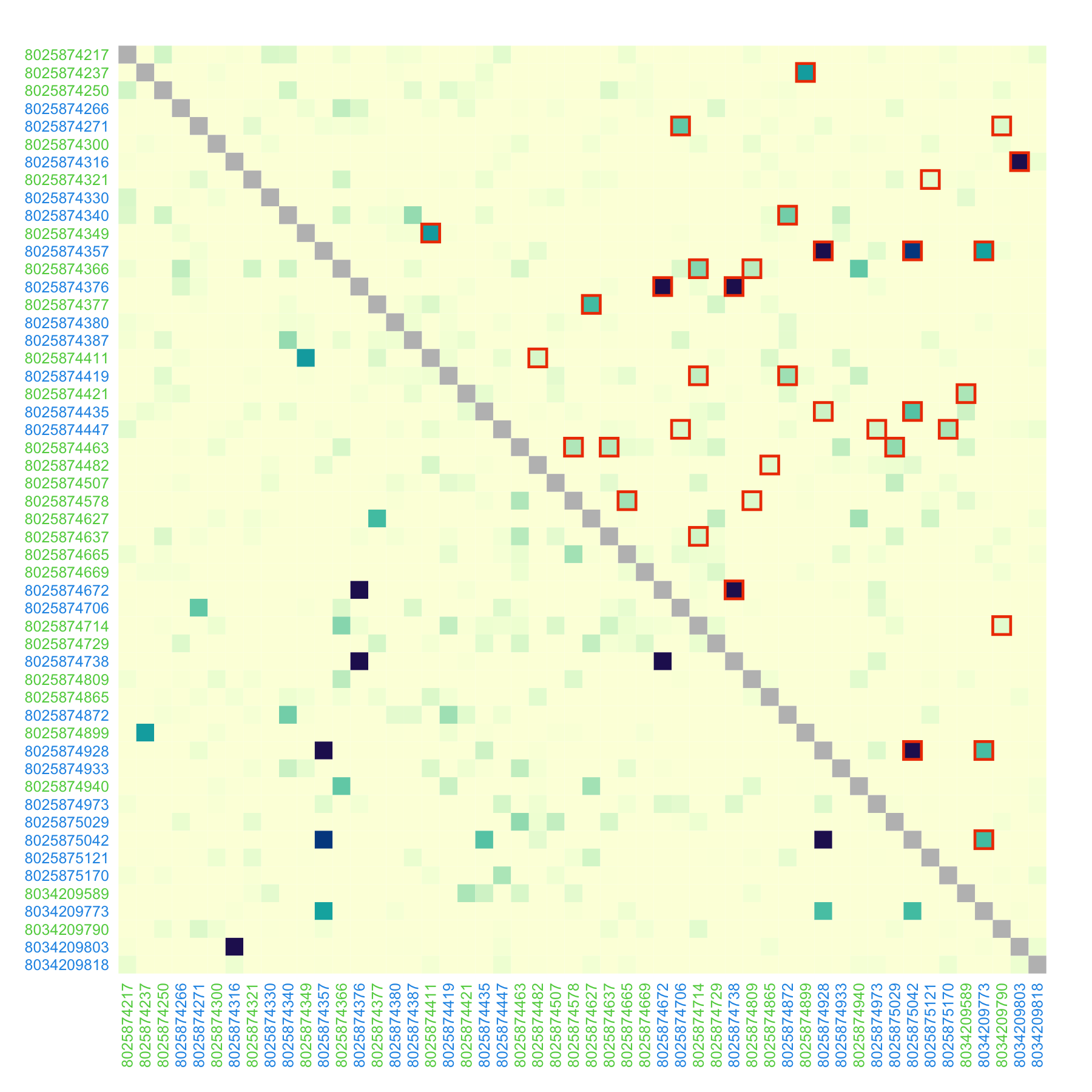For the sorted samples:

par(mar = c(1, 3, 3, 1))
nsmp  <- length(dsmp)
atsep <- cumsum(nsite)[-length(nsite)]
isig  <- which(dres[, , "p_value"] <= alpha, arr.ind = TRUE)
dmat  <- dres[, , "estimate"]
dmat[upper.tri(dmat)] <- t(dmat)[upper.tri(t(dmat))]
col_id <- rep(c("plum4", "lightblue4"), nsite)
plotRel(dmat, isig = rbind(isig, isig[, 2:1]), draw_diag = TRUE, alpha = alpha,
idlab = TRUE, side_id = c(2, 3), col_id = col_id, srt_id = c(25, 65))
abline(v = atsep, h = atsep, col = "gray45", lty = 5)For these symmetric distance measures, one of the triangles can be used to display other relevant information, such as p-values, geographic distance, or a number of non-missing loci between two samples. For that, use add = TRUE.

par(mfrow = c(1, 2), mar = c(1, 0, 1, 0.2))
plotRel(dres, draw_diag = TRUE, alpha = alpha)
mtext("p-values", 3, 0.2)
# p-values for upper triangle
pmat <- matrix(NA, length(dsmp), length(dsmp))
pmat[upper.tri(pmat)] <- t(log(dres[, , "p_value"]))[upper.tri(pmat)]
pmat[pmat == -Inf] <- min(pmat[is.finite(pmat)])#*1.2
plotRel(pmat, rlim = NULL, draw_diag = TRUE, col = hcl.colors(101, "Red-Purple"),
sig = FALSE, add = TRUE, col_diag = "white", border_diag = "gray45")
abline(v = atsep, h = atsep, col = "gray45", lty = 5)

# number of non-missing loci for upper triangle
dmiss <- lapply(dsmp, function(lst) sapply(lst, function(v) all(!v)))
nmat <- matrix(NA, nsmp, nsmp)
for (jsmp in 2:nsmp) {
for (ismp in 1:(jsmp - 1)) {
nmat[ismp, jsmp] <- sum(!dmiss[[ismp]] & !dmiss[[jsmp]])
}
}
nrng <- range(nmat, na.rm = TRUE)  #
par(mar = c(1, 0.2, 1, 0))
plotRel(dres, draw_diag = TRUE, alpha = alpha)
mtext("number of loci", 3, 0.2)
coln <- hcl.colors(diff(nrng)*2.4, "Purple-Blue", rev = TRUE)[1:(diff(nrng) + 1)]
plotRel(nmat, rlim = NA, col = coln, add = TRUE,
draw_diag = TRUE, col_diag = "gray45", border_diag = "white")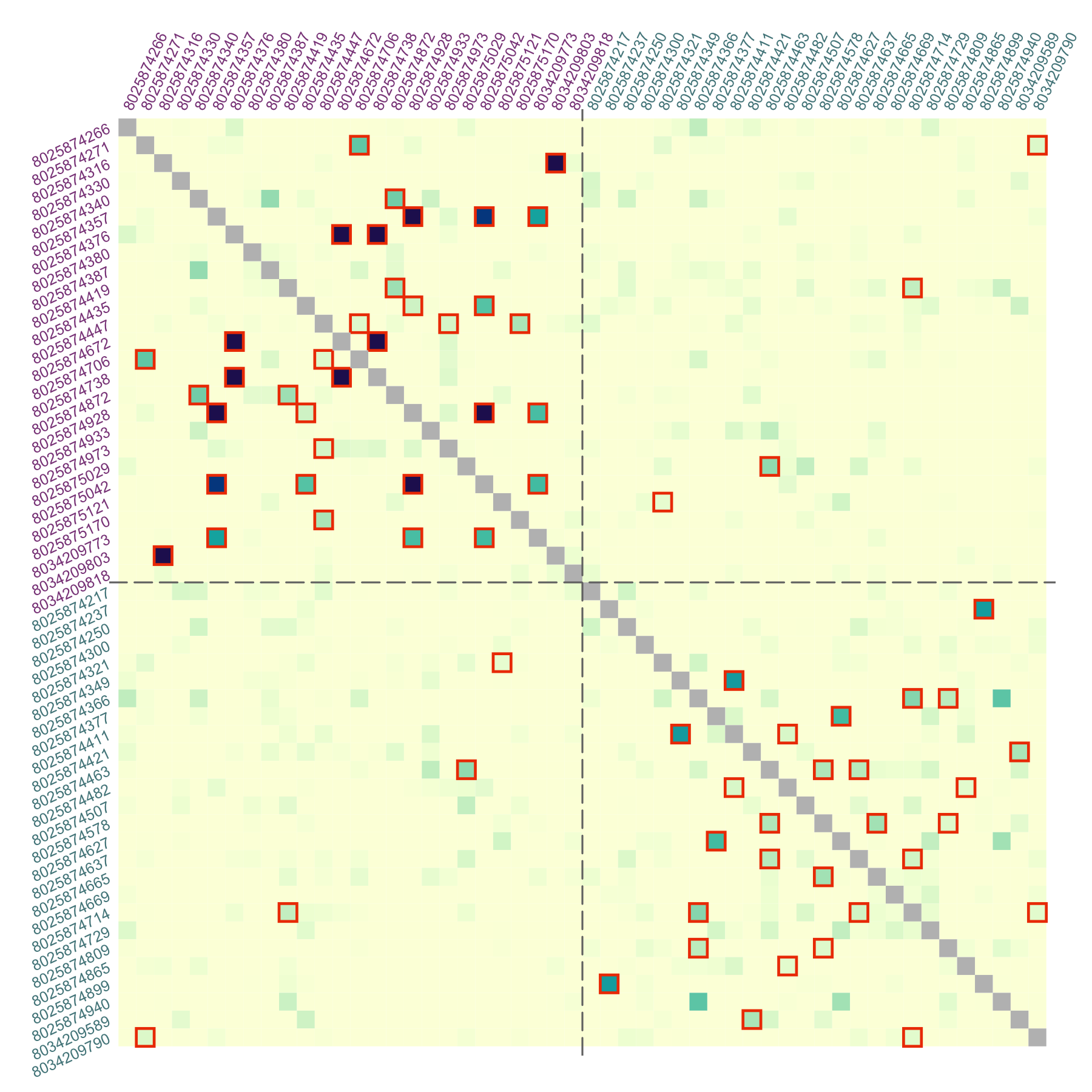par(pardef)

For reference, add a colorbar legend to the plot. It can be placed beside the main plot:

layout(matrix(1:2, 1), width = c(7, 1))
par(mar = c(1, 1, 2, 1))#, mgp = c(0, 0, 0.5))
plotRel(dmat, draw_diag = TRUE, isig = rbind(isig, isig[, 2:1]))
atclin <- cumsum(nsite) - nsite/2
abline(v = atsep, h = atsep, col = "gray45", lty = 5)
mtext(provinces, side = 3, at = atclin, line = 0.2)
mtext(provinces, side = 2, at = atclin, line = 0.2)
par(mar = c(1, 0, 2, 3))
plotColorbar()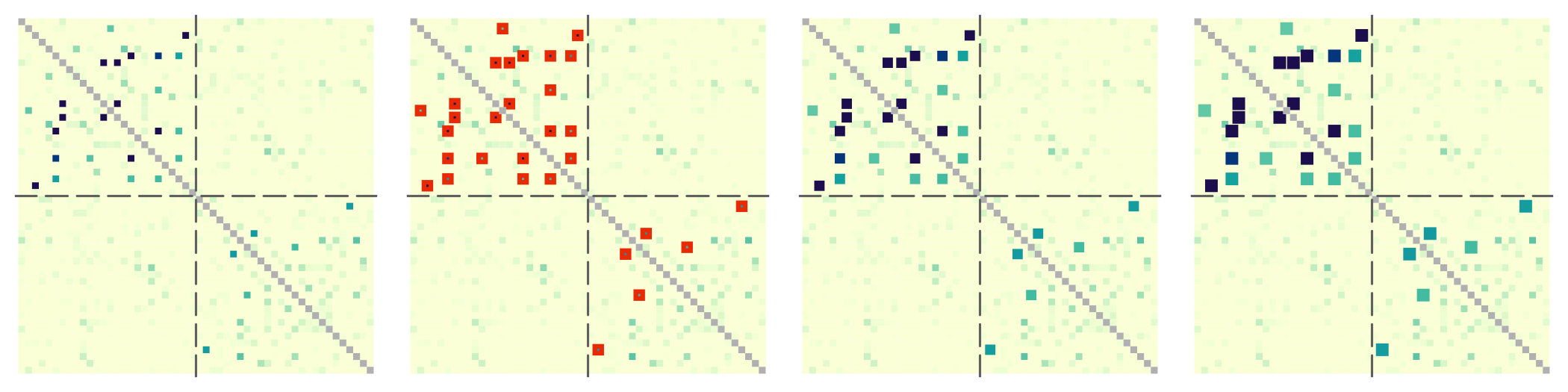par(pardef)

The colorbar can also be located in the empty space left by the triangular matrix. In the example below, we specify horizontal colorbar and provide custom tick mark locations:

# horizontal colorbar
par(mar = c(1, 1, 1, 3))
border_sig = "darkviolet"
plotRel(dres, draw_diag = TRUE, border_diag = border_sig, alpha = alpha,
border_sig = border_sig, lwd_sig = 2)
legend(32, 20, pch = 0, col = border_sig, pt.lwd = 2, pt.cex = 1.4,
box.col = "gray", legend = expression("significant at" ~~ alpha == 0.05))
text(1:nsmp + 0.3, 1:nsmp - 0.5, labels = names(dsmp), col = col_id, adj = 0,
cex = 0.55, xpd = TRUE)
par(fig = c(0.25, 1, 0.81, 0.92), mar = c(1, 1, 1, 1), new = TRUE)
plotColorbar(at = c(0.2, 0.4, 0.6, 0.8), horiz = TRUE)
ncol <- 301
lines(c(0, ncol, ncol, 0, 0), c(0, 0, 1, 1, 0), col = "gray")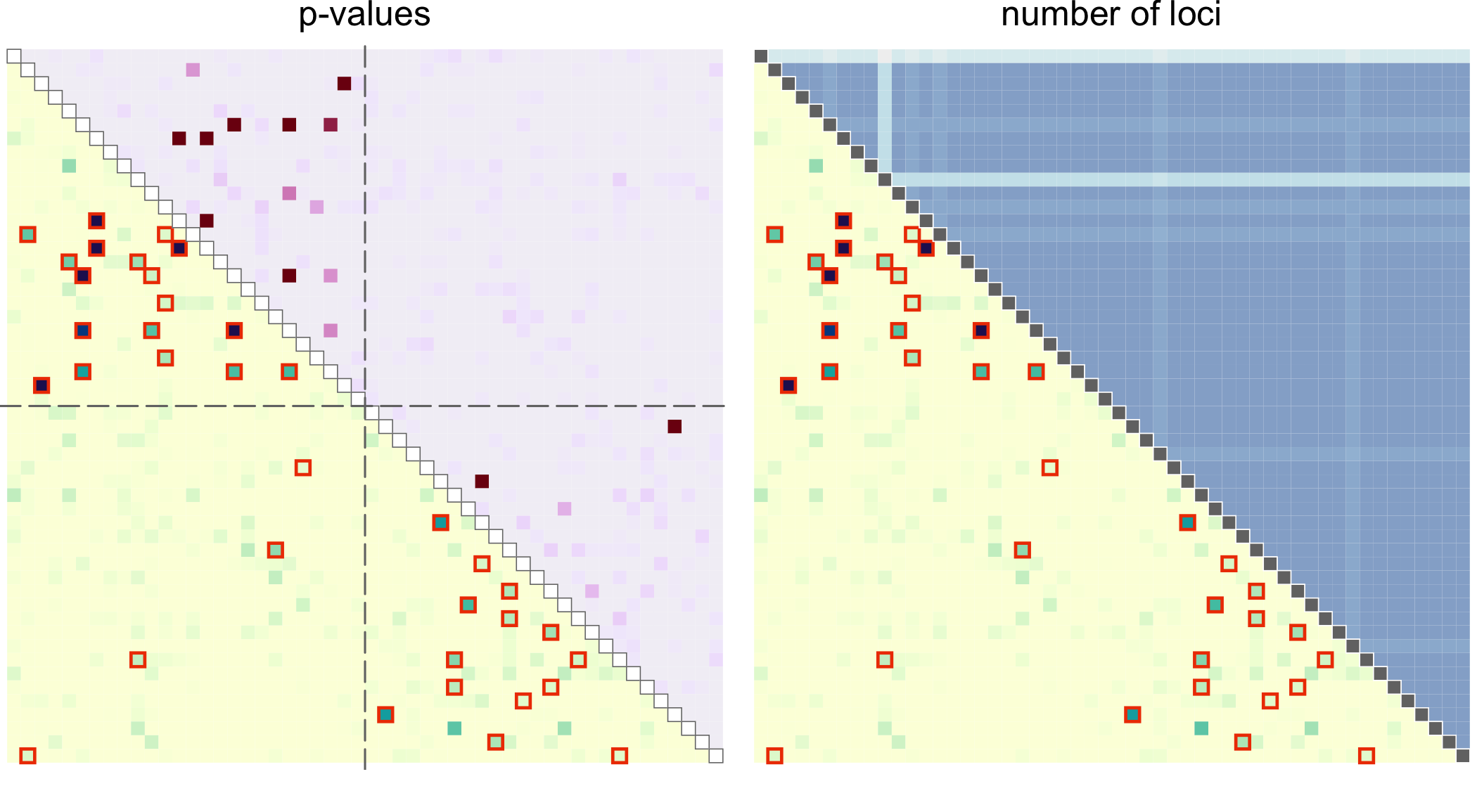par(pardef)

Examine pairs that are determined to be significantly related at the significance level more closely by allowing multiple pairs of strains to be related between two infections. Using ibdEstM, we also estimate the number of positively related pairs of strains and compare results yielded by a constrained model assuming (faster method) and without the constraint. In addition, we look at the estimates of overall relatedness.

# First, create a grid of r values to evaluate over
revals <- mapply(generateReval, 1:5, nr = c(1e3, 1e2, 32, 16, 12))
sig1 <- sig2 <- vector("list", nrow(isig))
for (i in 1:nrow(isig)) {
sig1[[i]] <- ibdEstM(dsmp[isig[i, ]], coi[isig[i, ]], afreq, Mmax = 5,
equalr = FALSE, reval = revals)
}
for (i in 1:nrow(isig)) {
sig2[[i]] <- ibdEstM(dsmp[isig[i, ]], coi[isig[i, ]], afreq, equalr = TRUE)
}
M1      <- sapply(sig1, function(r) sum(r > 0))
M2      <- sapply(sig2, length)
rtotal1 <- sapply(sig1, sum)
rtotal2 <- sapply(sig2, sum)

cor(M1, M2)
#>  0.9486851
cor(rtotal1, rtotal2)
#>  0.9998965

Create a list of significant pairs:

samples <- names(dsmp)
sig <- as.data.frame(isig, row.names = FALSE)
sig[c("id1", "id2")]         <- list(samples[isig[, 1]], samples[isig[, 2]])
sig[c("M1", "M2")]           <- list(M1, M2)
sig[c("rtotal1", "rtotal2")] <- list(round(rtotal1, 3), round(rtotal2, 3))
#>   row col        id1        id2 M1 M2 rtotal1 rtotal2
#> 1  14   2 8025874706 8025874271  1  1   0.410   0.412
#> 2  52   2 8034209790 8025874271  1  1   0.133   0.133
#> 3  25   3 8034209803 8025874316  1  1   1.000   1.000
#> 4  16   5 8025874872 8025874340  2  2   0.520   0.516
#> 5  17   6 8025874928 8025874357  1  1   1.000   1.000
#> 6  21   6 8025875042 8025874357  1  1   0.881   0.881

For full control, we can use the function ibdPair for any two samples and explore the outputs:

i <- 18
pair <- dsmp[isig[i, ]]
coii <-  coi[isig[i, ]]

res1 <- ibdPair(pair, coii, afreq, M = M1[i], pval = TRUE, equalr = FALSE,
reval = revals[[M1[i]]])
res2 <- ibdPair(pair, coii, afreq, M = M2[i], pval = TRUE, equalr = TRUE,
confreg = TRUE, llik = TRUE, reval = revals[])

res1$rhat # estimate with equalr = FALSE #>  0.31 1.00 rep(res2$rhat, M2[i])                  # estimate with equalr = TRUE
#>  0.673 0.673

When llik = TRUE, output includes log-likelihood (for a single parameter if we use equalr = TRUE), which provides the basis for statistical inference:

CI     <- range(res2$confreg) llikCI <- max(res2$llik) - qchisq(1 - alpha, df = 1)/2
llrng  <- range(res2$llik[is.finite(res2$llik)])
yCI <- llrng + diff(llrng)*0.25
yLR <- (res2$llik + max(res2$llik))/2

par(mar = c(3, 2.5, 2, 0.1), mgp = c(1.5, 0.3, 0))
plot(revals[], res2$llik, type = "l", xlab = "r", ylab = "log-likelihood", yaxt = "n")#, tck = -0.01) abline(v = res2$rhat, lty = 1, col = cols)
abline(h = c(max(res2$llik), llikCI, res2$llik[]), lty = 2, col = cols)
abline(v = CI, col = cols, lty = 5)
arrows(CI, yCI, CI, yCI, angle = 20, length = 0.1, code = 3,
col = cols)
arrows(0.15, res2$llik, 0.15, max(res2$llik), angle = 20, length = 0.1,
code = 3, col = cols)
text(0.165, yLR, adj = 0, "0.5 LR statistic", col = cols)
text(mean(CI), yCI + 0.05*diff(llrng), "confidence interval", col = cols)
text(res2\$rhat - 0.025, yLR, "MLE", col = cols, srt = 90)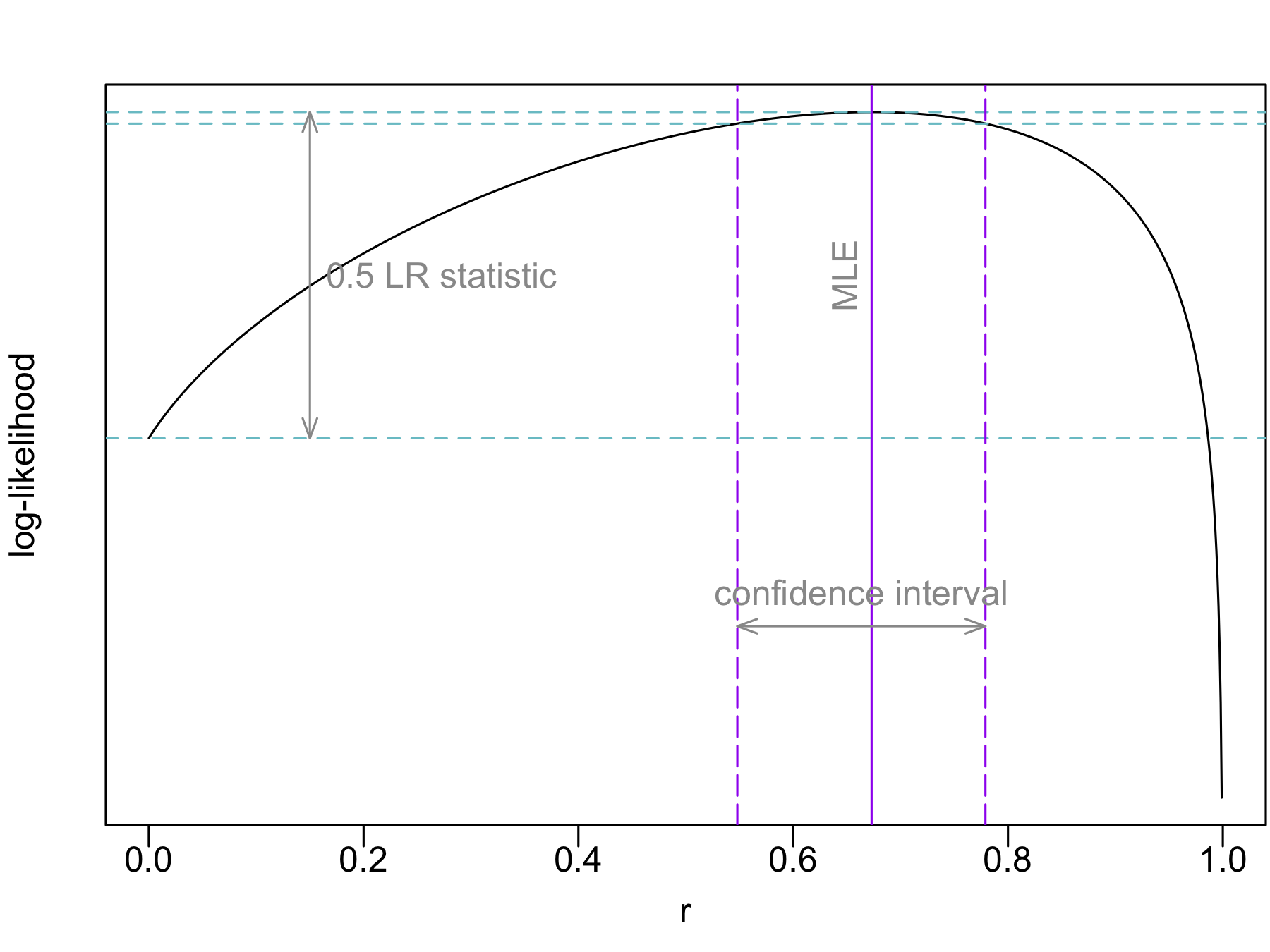par(pardef)
1.
Gerlovina I, Gerlovin B, Rodríguez-Barraquer I, Greenhouse B. Dcifer: an IBD-based method to calculate genetic distance between polyclonal infections. Genetics. 2022 Aug;222(2).
2.
Tessema SK, Hathaway NJ, Teyssier NB, Murphy M, Chen A, Aydemir O, et al. Sensitive, highly multiplexed sequencing of microhaplotypes from the Plasmodium falciparum heterozygome. The Journal of infectious diseases. 2022;225(7):1227–37.